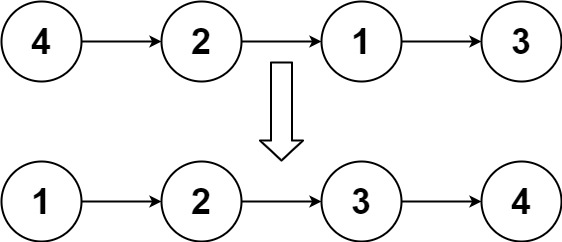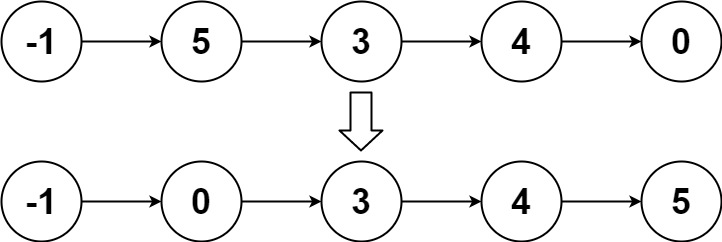# LeetCode | 148. Sort List

Given the head of a linked list, return the list after sorting it in ascending order.

Follow up: Can you sort the linked list in O(n logn) time and O(1) memory (i.e. constant space)?

Example 1:Input: head = [4,2,1,3]
Output: [1,2,3,4]


Example 2:Input: head = [-1,5,3,4,0]
Output: [-1,0,3,4,5]


Example 3:

Input: head = []
Output: []


Constraints:

• The number of nodes in the list is in the range [0, 5 * 104].
• -105 <= Node.val <= 105

/**
* struct ListNode {
*     int val;
*     ListNode *next;
*     ListNode(int x) : val(x), next(NULL) {}
* };
*/
class Solution {
public:
void swap(int& a, int& b) {
int tmp = a;
a = b;
b = tmp;
return;
}
ListNode* getPartition(ListNode* head, ListNode* end) {
while(cur != end)
{
if(cur->val < key->val)
{
p = p->next;
swap(cur->val, p->val);
}
cur = cur->next;
}
swap(key->val, p->val);
return p;
}
void sort(ListNode* head, ListNode* end) {
return;
sort(partition->next, end);
}
}
};

07-11510001-10638
08-065244
02-26285
04-18391
09-20450
10-04352
03-20513
03-202941
02-22317